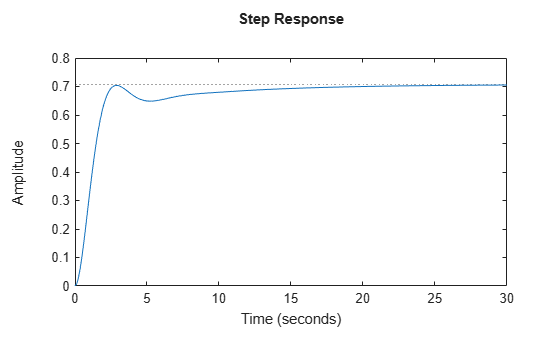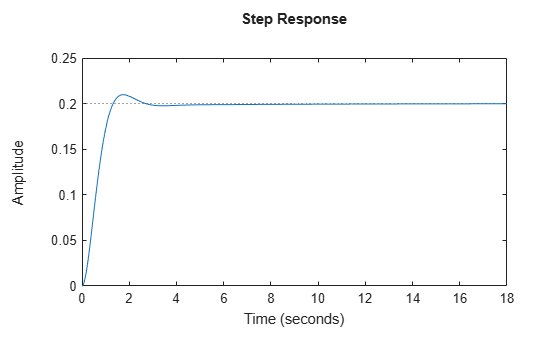# lqr

## Syntax

``[K,S,P] = lqr(sys,Q,R,N)``
``[K,S,P] = lqr(A,B,Q,R,N)``

## Description

example

````[K,S,P] = lqr(sys,Q,R,N)` calculates the optimal gain matrix `K`, the solution `S` of the associated algebraic Riccati equation, and the closed-loop poles `P` for the continuous-time or discrete-time state-space model `sys`. `Q` and `R` are the weight matrices for states and inputs, respectively. The cross term matrix `N` is set to zero when omitted.```

example

````[K,S,P] = lqr(A,B,Q,R,N)` calculates the optimal gain matrix `K`, the solution `S` of the associated algebraic Riccati equation and the closed-loop poles `P` using the continuous-time state-space matrices `A` and `B`.```

## Examples

collapse all

`pendulumModelCart.mat` contains the state-space model of an inverted pendulum on a cart where the outputs are the cart displacement `x` and the pendulum angle $\theta$. The control input `u` is the horizontal force on the cart.

`$\begin{array}{l}\left[\begin{array}{c}\underset{}{\overset{˙}{x}}\\ \underset{}{\overset{¨}{x}}\\ \underset{}{\overset{˙}{\theta }}\\ \underset{}{\overset{¨}{\theta }}\end{array}\right]\phantom{\rule{0.2777777777777778em}{0ex}}=\phantom{\rule{0.2777777777777778em}{0ex}}\left[\begin{array}{cccc}0& 1& 0& 0\\ 0& -0.1& 3& 0\\ 0& 0& 0& 1\\ 0& -0.5& 30& 0\end{array}\right]\left[\begin{array}{c}x\\ \underset{}{\overset{˙}{x}}\\ \theta \\ \underset{}{\overset{˙}{\theta }}\end{array}\right]+\left[\begin{array}{c}0\\ 2\\ 0\\ 5\end{array}\right]u\\ \phantom{\rule{0.2777777777777778em}{0ex}}\phantom{\rule{0.2777777777777778em}{0ex}}\phantom{\rule{0.2777777777777778em}{0ex}}\phantom{\rule{0.2777777777777778em}{0ex}}\phantom{\rule{0.2777777777777778em}{0ex}}y\phantom{\rule{0.2777777777777778em}{0ex}}=\phantom{\rule{0.2777777777777778em}{0ex}}\left[\begin{array}{cccc}1& 0& 0& 0\\ 0& 0& 1& 0\end{array}\right]\left[\begin{array}{c}x\\ \underset{}{\overset{˙}{x}}\\ \theta \\ \underset{}{\overset{˙}{\theta }}\end{array}\right]+\left[\begin{array}{c}0\\ 0\end{array}\right]u\end{array}$`

First, load the state-space model `sys` to the workspace.

`load('pendulumCartModel.mat','sys')`

Since the outputs are `x` and $\theta$, and there is only one input, use Bryson's rule to determine `Q` and `R`.

```Q = [1,0,0,0;... 0,0,0,0;... 0,0,1,0;... 0,0,0,0]; R = 1;```

Find the gain matrix `K` using `lqr`. Since `N` is not specified, `lqr` sets `N` to 0.

`[K,S,P] = lqr(sys,Q,R)`
```K = 1×4 -1.0000 -1.7559 16.9145 3.2274 ```
```S = 4×4 1.5346 1.2127 -3.2274 -0.6851 1.2127 1.5321 -4.5626 -0.9640 -3.2274 -4.5626 26.5487 5.2079 -0.6851 -0.9640 5.2079 1.0311 ```
```P = 4×1 complex -0.8684 + 0.8523i -0.8684 - 0.8523i -5.4941 + 0.4564i -5.4941 - 0.4564i ```

Although Bryson's rule usually provides satisfactory results, it is often just the starting point of a trial-and-error iterative design procedure to tune your closed-loop system response based on the design requirements.

`aircraftPitchModel.mat` contains the state-space matrices of an aircraft where the input is the elevator deflection angle $\delta$ and the output is the aircraft pitch angle $\theta$.

`$\begin{array}{l}\left[\begin{array}{c}\underset{}{\overset{˙}{\alpha }}\\ \underset{}{\overset{˙}{q}}\\ \underset{}{\overset{˙}{\theta }}\end{array}\right]\phantom{\rule{0.2777777777777778em}{0ex}}=\phantom{\rule{0.2777777777777778em}{0ex}}\left[\begin{array}{ccc}-0.313& 56.7& 0\\ -0.0139& -0.426& 0\\ 0& 56.7& 0\end{array}\right]\left[\begin{array}{c}\alpha \\ q\\ \theta \end{array}\right]+\left[\begin{array}{c}0.232\\ 0.0203\\ 0\end{array}\right]\left[\delta \right]\\ \phantom{\rule{0.2777777777777778em}{0ex}}\phantom{\rule{0.2777777777777778em}{0ex}}\phantom{\rule{0.2777777777777778em}{0ex}}\phantom{\rule{0.2777777777777778em}{0ex}}\phantom{\rule{0.2777777777777778em}{0ex}}y\phantom{\rule{0.2777777777777778em}{0ex}}=\phantom{\rule{0.2777777777777778em}{0ex}}\left[\begin{array}{ccc}0& 0& 1\end{array}\right]\left[\begin{array}{c}\alpha \\ q\\ \theta \end{array}\right]+\left[0\right]\left[\delta \right]\end{array}$`

For a step reference of 0.2 radians, consider the following design criteria:

• Rise time less than 2 seconds

• Settling time less than 10 seconds

• Steady-state error less than 2%

Load the model data to the workspace.

`load('aircraftPitchModel.mat')`

Define the state-cost weighted matrix `Q` and the control weighted matrix `R`. Generally, you can use Bryson's Rule to define your initial weighted matrices `Q` and `R`. For this example, consider the output vector `C` along with a scaling factor of 5 for matrix `Q` and choose `R` as 1. `R` is a scalar since the system has only one input.

`R = 1`
```R = 1 ```
`Q1 = 2*C'*C`
```Q1 = 3×3 0 0 0 0 0 0 0 0 2 ```

Compute the gain matrix using `lqr`.

`[K1,S1,P1] = lqr(A,B,Q1,R);`

Check the closed-loop step response with the generated gain matrix `K1`.

```sys1 = ss(A-B*K1,B,C,D); step(sys1)```Since this response does not meet the design goals, increase the scaling factor to 25, compute the gain matrix `K2,` and check the closed-loop step response for gain matrix `K2`.

`Q2 = 25*C'*C`
```Q2 = 3×3 0 0 0 0 0 0 0 0 25 ```
```[K2,S2,P2] = lqr(A,B,Q2,R); sys2 = ss(A-B*K2,B,C,D); step(sys2)```In the closed-loop step response plot, the rise time, settling time, and steady-state error meet the design goals.

## Input Arguments

collapse all

Dynamic system model, specified as an `ss` model object.

State matrix, specified as an `n`-by-`n` matrix, where `n` is the number of states.

Input-to-state matrix, specified as an `n`-by-`m` input-to-state matrix, where `m` is the number of inputs.

State-cost weighted matrix, specified as an `n`-by-`n` matrix, where `n` is the number of states. You can use Bryson's rule to set the initial values of `Q` given by:

Here, `n` is the number of states.

Input-cost weighted matrix, specified as a scalar or a matrix of the same size as `D'D`. Here, `D` is the feed-through state-space matrix. You can use Bryson's rule to set the initial values of `R` given by:

Here, `m` is the number of inputs.

Optional cross term matrix, specified as a matrix. If `N` is not specified, then `lqr` sets `N` to 0 by default.

## Output Arguments

collapse all

Optimal gain of the closed-loop system, returned as a row vector of size `n`, where `n` is the number of states.

Solution of the associated algebraic Riccati equation, returned as an `n`-by-`n` matrix, where `n` is the number of states. In other words, `S` is the same dimension as state-space matrix `A`. For more information, see `icare` and `idare`.

Poles of the closed-loop system, returned as a column vector of size `n`, where `n` is the number of states.

## Limitations

The input data must satisfy the following conditions:

• The pair `A` and `B` must be stabilizable.

• `[Q,N;N',R]` must be nonnegative definite.

• `R>0` and $Q-N{R}^{-1}{N}^{T}\ge 0$.

• $\left(Q-N{R}^{-1}{N}^{T},\text{\hspace{0.17em}}A-B{R}^{-1}{N}^{T}\right)$ has no unobservable mode on the imaginary axis (or unit circle in discrete time).

## Tips

• `lqr` supports descriptor models with nonsingular `E`. The output `S` of `lqr` is the solution of the algebraic Riccati equation for the equivalent explicit state-space model:

`$\frac{dx}{dt}={E}^{-1}Ax+{E}^{-1}Bu$`

## Algorithms

For continuous-time systems, `lqr` computes the state-feedback control $u=-Kx$ that minimizes the quadratic cost function

`$J\left(u\right)={\int }_{0}^{\infty }\left({x}^{T}Qx+{u}^{T}Ru+2{x}^{T}Nu\right)dt$`

subject to the system dynamics $\stackrel{˙}{x}=Ax+Bu$.

In addition to the state-feedback gain `K`, `lqr` returns the solution `S` of the associated algebraic Riccati equation

and the closed-loop poles . The gain matrix `K` is derived from `S` using

`$K={R}^{-1}\left({B}^{T}S+{N}^{T}\right).$`

For discrete-time systems, `lqr` computes the state-feedback control ${u}_{n}=-K{x}_{n}$ that minimizes

`$J=\sum _{n=0}^{\infty }\left\{{x}^{T}Qx+{u}^{T}Ru+2{x}^{T}Nu\right\}$`

subject to the system dynamics ${x}_{n+1}=A{x}_{n}+B{u}_{n}$.

In all cases, when you omit the cross term matrix `N`, `lqr` sets `N` to 0.